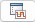# Prove System-Level Properties Using Verification Model

This example shows how you can use a verification model to prove system-level properties.

### When to Use a Verification Model for Property Proving

If your model has system-wide properties that affect the behavior of the model, you might want to prove the properties without changing the design model. To do this, you create a verification model that includes:

• Model block that references the design model

• One or more verification subsystems that define the properties and any required constraints

The design model `sldvdemo_sbr_design` models the logic for a seat belt reminder light. If the ignition is turned on, the seat belts are unfastened, and the car exceeds a certain speed, the seat belt reminder light turns on.

The `sldvdemo_sbr_verification` model is a verification model that defines some constraints and verifies the properties in the `sldvdemo_sbr_design` model. The Model block in the verification model references the design model, so that the verification logic exists only in the verification model.

The` sldvdemo_sbr_verification` model contains a property that is falsified, because a constraint is disabled. In the `sldvdemo_sbl_verification_fixed` model, the constraint is enabled and all the properties are proven valid.

### Understand the Verification Model

Take these steps to understand how the verification model works:

1. Open the verification model:

`open_system('sldvdemo_sbr_verification')`

The Design Model block is a Model block that references `sldvdemo_sbr_design`. The SBR Stateflow® chart in the design model assumes that the KEY input is initially 0.

2. Open the Safety Properties subsystem that specifies the properties of the design model that you want to prove.

This subsystem contains a MATLAB Function block called MATLAB Property. The code in this block specifies the property that the seat belt reminder should be on when the ignition is on, the seat belt is not fastened, and the speed is less than 15:

3. Close the Safety Properties subsystem.

4. Open the Input Constraints subsystem.

This subsystem defines the following constraints:

• The key can have three positions: 0, 1, 2

• The speed is constrained to fall between 10 and 30.

• The key must start at 0 and can only change by one increment at a time. For example, the key can change from 0 to 1 or 1 to 2, but not from 0 to 2. In this verification model, this constraint is not enabled.

5. Close the Input Constraints subsystem, but keep the `sldvdemo_sbr_verification` model open.

### Prove the Properties of the Design Model

Analyze the `sldvdemo_sbr_verification` model to prove the properties:

1. In the `sldvdemo_sbr_verification` model window, to start the analysis, double-click the Run button to start the analysis.

When the analysis completes, the Simulink® Design Verifier[tm] log window indicates that one objective is falsified - needs simulation. For more information, see Objectives Falsified - Needs Simulation.

2. To see which objective was falsified, click Highlight analysis results on model.

The Safety Properties subsystem is highlighted in orange.

3. Open the Safety Properties subsystem and click the MATLAB Property block.

The Simulink® Design Verifier™ Results window indicates that the statement `sldv.prove(implies(activeCond,SeatBeltIcon)) `was false during at least one time step.4. Click View counterexample to see the signal values that violated this property.

The Signal Builder block opens with the counterexample. The KEY input was initially 2, which is invalid.

To validate the property specified in the Safety Properties subsystem, you have to make sure that the initial value of KEY is 0.

### Fix the Verification Model

The Input Constraints subsystem in the verification model contained three constraints. The third constraint, which requires that the initial value of KEY be 0, and that KEY can only change in increments of 1, is disabled.To see how this property is validated when you enable the third constraint:

1. In the `sldvdemo_sbr_verification` model, click Open Fixed Model.

The `sldvdemo_sbr_verification_fixed` verification model opens.

2. Open the Input Constraints subsystem.

This third constraint is now enabled so that KEY has an initial value of 0 and changes in increments of 1.

3. Close the Input Constraints subsystem.

4. In the `sldvdemo_sbr_verification_fixed `model, to start the analysis, double-click the Run block.

The analysis proves the validity of the property.